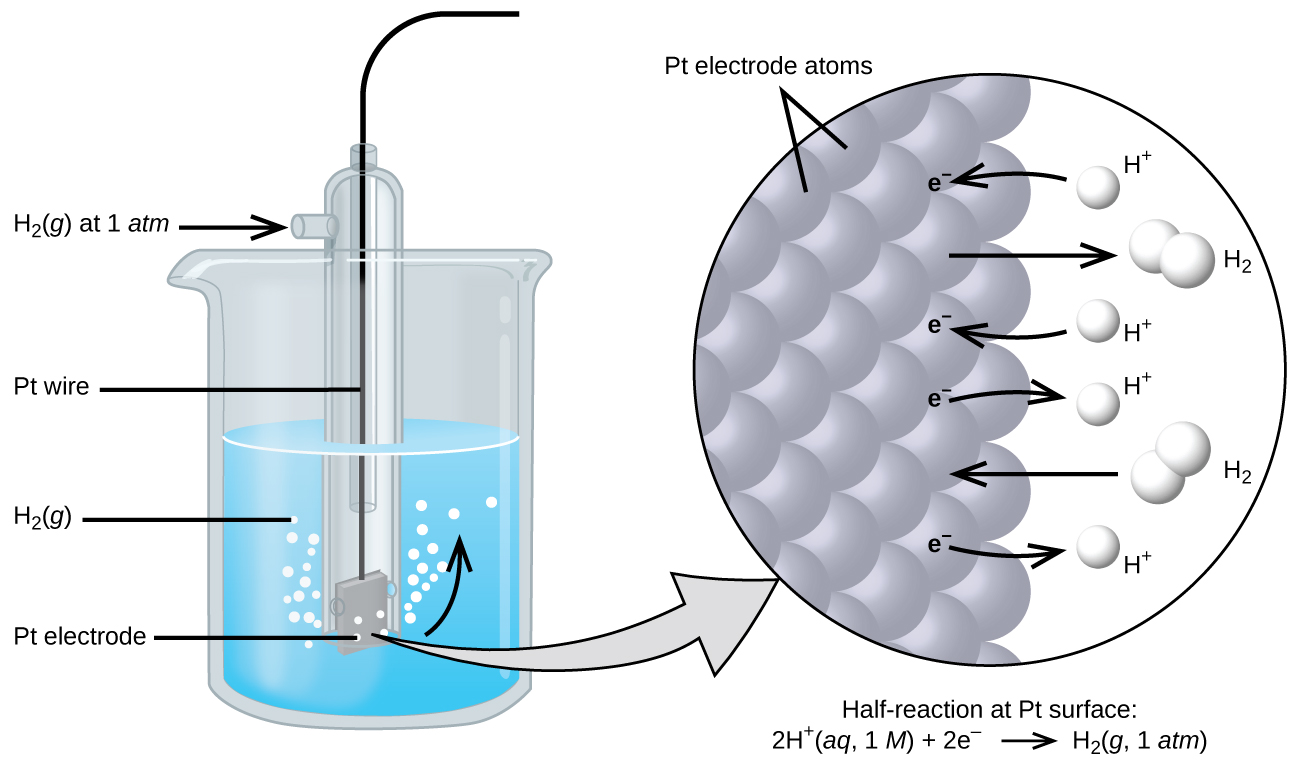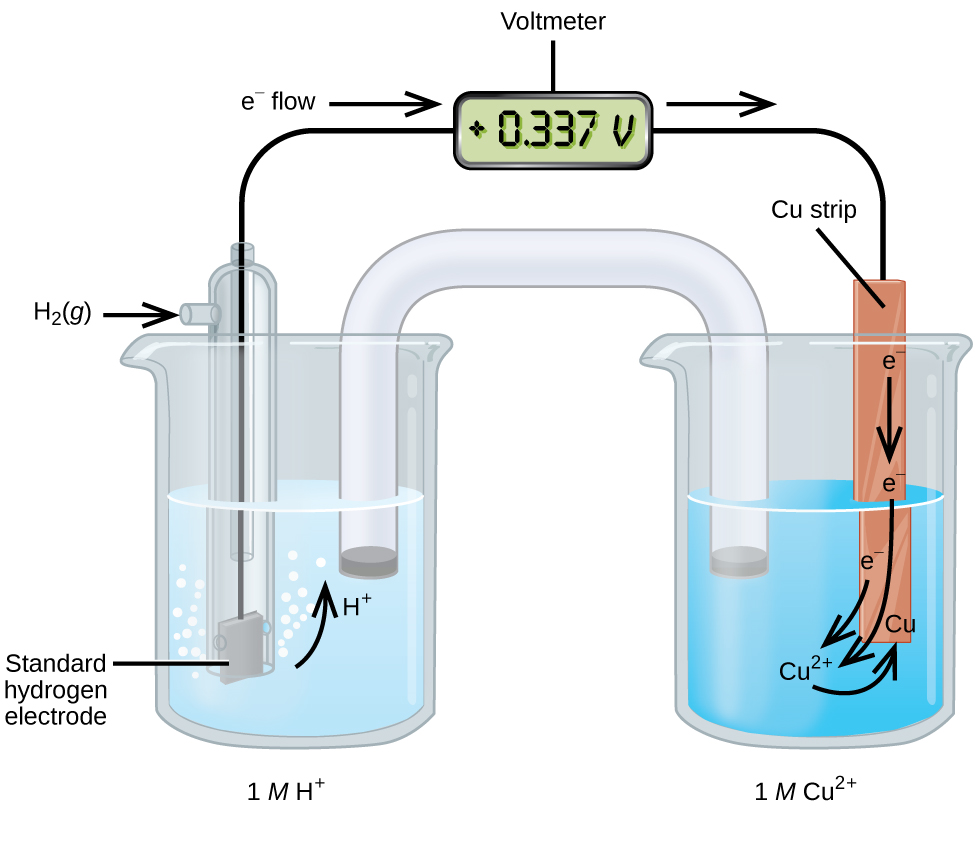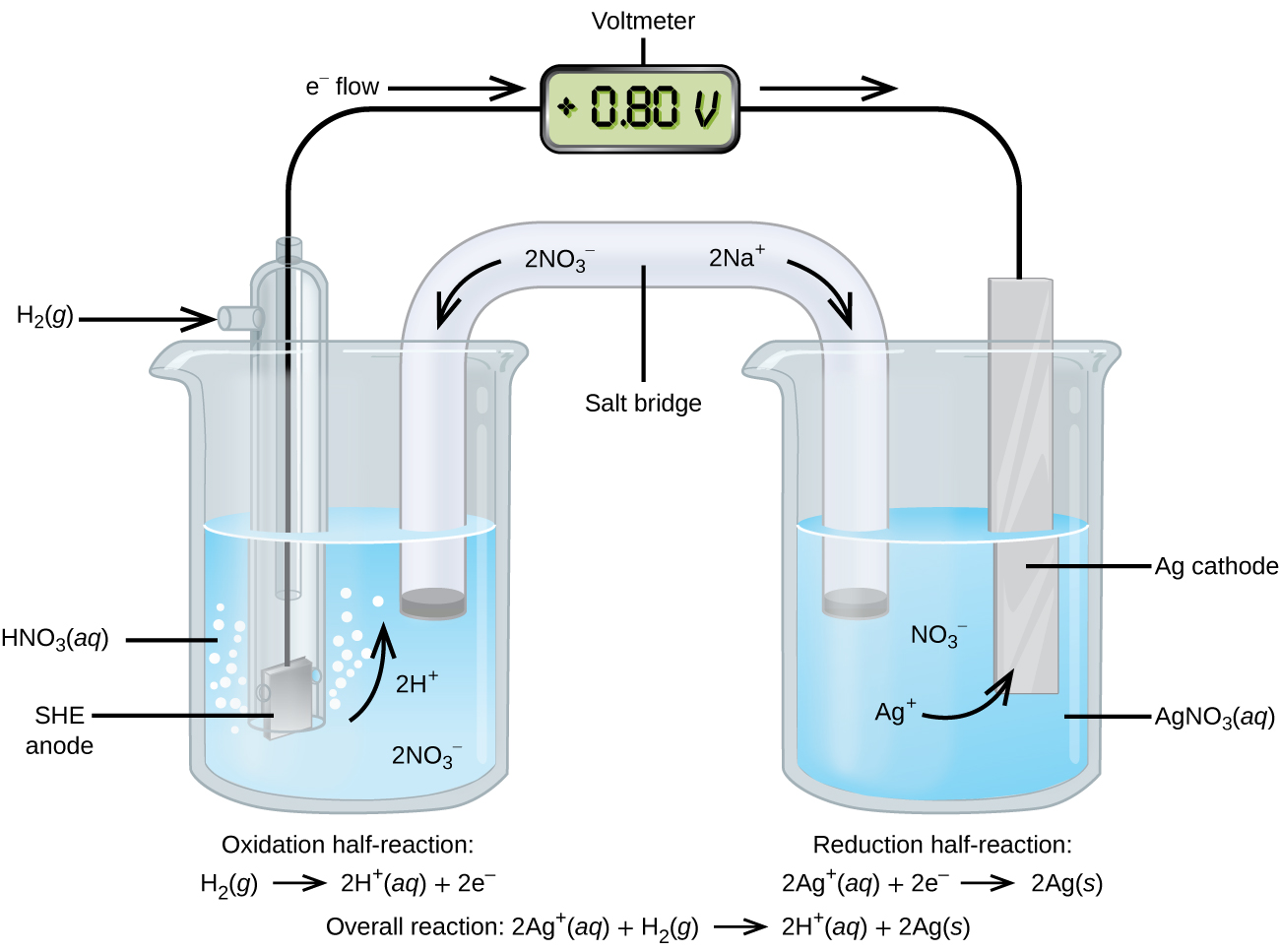This book is archived and will be removed July 6, 2022. Please use the updated version.

Chapter 17. Electrochemistry

# 17.3 Standard Reduction Potentials

### Learning Objectives

By the end of this section, you will be able to:
• Determine standard cell potentials for oxidation-reduction reactions
• Use standard reduction potentials to determine the better oxidizing or reducing agent from among several possible choices

The cell potential in Chapter 17.2 Galvanic Cells (+0.46 V) results from the difference in the electrical potentials for each electrode. While it is impossible to determine the electrical potential of a single electrode, we can assign an electrode the value of zero and then use it as a reference. The electrode chosen as the zero is shown in Figure 1 and is called the standard hydrogen electrode (SHE). The SHE consists of 1 atm of hydrogen gas bubbled through a 1 M HCl solution, usually at room temperature. Platinum, which is chemically inert, is used as the electrode. The reduction half-reaction chosen as the reference is

$2\text{H}^{+}(aq\text{,}\;1\;M)\;+\;2\text{e}^{-}\;{\rightleftharpoons}\;\text{H}_2(g\text{,}\;1\;\text{atm})\;\;\;\;\;\;E^{\circ} = 0\;\text{V}$

E° is the standard reduction potential. The superscript “°” on the E denotes standard conditions (1 bar or 1 atm for gases, 1 M for solutes). The voltage is defined as zero for all temperatures.Figure 1. Hydrogen gas at 1 atm is bubbled through 1 M HCl solution. Platinum, which is inert to the action of the 1 M HCl, is used as the electrode. Electrons on the surface of the electrode combine with H+ in solution to produce hydrogen gas.

A galvanic cell consisting of a SHE and Cu2+/Cu half-cell can be used to determine the standard reduction potential for Cu2+ (Figure 2). In cell notation, the reaction is

$\text{Pt}(s){\mid}\text{H}_2(g\text{,\;}1\;\text{atm}){\mid}\text{H}^{+}(aq\text{,}\;1\;M){\parallel}\text{Cu}^{2+}(aq\text{,}\;1\;M){\mid}\text{Cu}(s)$

Electrons flow from the anode to the cathode. The reactions, which are reversible, are

$\begin{array}{lr @{{}\longrightarrow{}} l} \text{Anode\;(oxidation):} & \text{H}_2(g) & 2\text{H}^{+}(aq)\;+\;2\text{e}^{-} \\[0.5em] \text{Cathode\;(reduction):} & \text{Cu}^{2+}(aq)\;+\;2\text{e}^{-} & \text{Cu}(s) \\[0.5em] \hline \\[-0.25em] \text{Overall:} & \text{Cu}^{2+}(aq)\;+\;\text{H}_2(g) & 2\text{H}^{+}(aq)\;+\;\text{Cu}(s) \end{array}$

The standard reduction potential can be determined by subtracting the standard reduction potential for the reaction occurring at the anode from the standard reduction potential for the reaction occurring at the cathode. The minus sign is necessary because oxidation is the reverse of reduction.

$E_{\text{cell}}^{\circ} = E_{\text{cathode}}^{\circ}\;-\;E_{\text{anode}}^{\circ}$
$+0.34\;\text{V} = E_{\text{Cu}^{2+}/\text{Cu}}^{\circ}\;-\;E_{\text{H}^{+}/\text{H}_2}^{\circ} = E_{\text{Cu}^{2+}/\text{Cu}}^{\circ}\;-\;0 = E_{\text{Cu}^{2+}/\text{Cu}}^{\circ}$Figure 2. A galvanic cell can be used to determine the standard reduction potential of Cu2+.

Using the SHE as a reference, other standard reduction potentials can be determined. Consider the cell shown in Figure 3, where

$\text{Pt}(s){\mid}\text{H}_2(g\text{,\;}1\;\text{atm}){\mid}\text{H}^{+}(aq\text{,\;}1\;M){\parallel}\text{Ag}^{+}(aq\text{,\;}1\;M){\mid}\text{Ag}(s)$

Electrons flow from left to right, and the reactions are

$\begin{array}{lr @{{}\longrightarrow{}} l} \text{anode\;(oxidation):} & \text{H}_2(g) & 2\text{H}^{+}(aq)\;+\;2\text{e}^{-} \\[0.5em] \text{cathode\;(reduction):} & 2\text{Ag}^{+}(aq)\;+\;2\text{e}^{-} & 2\text{Ag}(s) \\[0.5em] \hline \\[-0.25em] \text{overall:} & 2\text{Ag}^{+}(aq)\;+\;\text{H}_2(g) & 2\text{H}^{+}(aq)\;+\;2\text{Ag}(s) \end{array}$

The standard reduction potential can be determined by subtracting the standard reduction potential for the reaction occurring at the anode from the standard reduction potential for the reaction occurring at the cathode. The minus sign is needed because oxidation is the reverse of reduction.

$E_{\text{cell}}^{\circ} = E_{\text{cathode}}^{\circ}\;-\;E_{\text{anode}}^{\circ}$
$+0.80\;\text{V} = E_{\text{Ag}^{+}/\text{Ag}}^{\circ}\;-\;E_{\text{H}^{+}/\text{H}_2}^{\circ} = E_{\text{Ag}^{+}/\text{Ag}}^{\circ}\;-\;0 = E_{\text{Ag}^{+}/\text{Ag}}^{\circ}$

It is important to note that the potential is not doubled for the cathode reaction.

The SHE is rather dangerous and rarely used in the laboratory. Its main significance is that it established the zero for standard reduction potentials. Once determined, standard reduction potentials can be used to determine the standard cell potential, $E_{\text{cell}}^{\circ}$, for any cell. For example, for the cell shown in Figure 2 in Chapter 17.2 Galvanic Cells,

$\text{Cu}(s){\mid}\text{Cu}^{2+}(aq\text{,\;}1\;M){\parallel}\text{Ag}^{+}(aq\text{,\;}1\;M){\mid}\text{Ag}(s)$
$\begin{array}{lr @{{}\longrightarrow{}} l} \text{anode\;(oxidation):} & \text{Cu}(s) & \text{Cu}^{2+}(aq)\;+\;2\text{e}^{-} \\[0.5em] \text{cathode\;(reduction):} & 2\text{Ag}^{+}(aq)\;+\;2\text{e}^{-} & 2\text{Ag}(s) \\[0.5em] \hline \\[-0.25em] \text{overall:} & \text{Cu}(s)\;+\;2\text{Ag}^{+}(aq) & \text{Cu}^{2+}(aq)\;+\;2\text{Ag}(s) \end{array}$
$E_{\text{cell}}^{\circ} = E_{\text{cathode}}^{\circ}\;-\;E_{\text{anode}}^{\circ} = E_{\text{Ag}^{+}/\text{Ag}}^{\circ}\;-\;E_{\text{Cu}^{2+}/\text{Cu}}^{\circ} = 0.80\;\text{V}\;-\;0.34\;\text{V} = 0.46\;\text{V}$

Again, note that when calculating $E_{\text{cell}}^{\circ}$, standard reduction potentials always remain the same even when a half-reaction is multiplied by a factor. Standard reduction potentials for selected reduction reactions are shown in Table 2. A more complete list is provided in Appendix L.Figure 3. A galvanic cell can be used to determine the standard reduction potential of Ag+. The SHE on the left is the anode and assigned a standard reduction potential of zero.
Half-Reaction E° (V)
$\text{F}_2(g)\;+\;2\text{e}^{-}\;{\longrightarrow}\;2\text{F}^{-}(aq)$ +2.866
$\text{PbO}_2(s)\;+\;\text{SO}_4^{\;\;2-}(aq)\;+\;4\text{H}^{+}(aq)\;+\;2\text{e}^{-}\;{\longrightarrow}\;\text{PbSO}_4(s)\;+\;2\text{H}_2\text{O}(l)$ +1.69
$\text{MnO}_4^{\;\;-}(aq)\;+\;8\text{H}^{+}(aq)\;+\;5\text{e}^{-}\;{\longrightarrow}\;\text{Mn}^{2+}(aq)\;+\;4\text{H}_2\text{O}(l)$ +1.507
$\text{Au}^{3+}(aq)\;+\;3\text{e}^{-}\;{\longrightarrow}\;\text{Au}(s)$ +1.498
$\text{Cl}_2(g)\;+\;2\text{e}^{-}\;{\longrightarrow}\;2\text{Cl}^{-}(aq)$ +1.35827
$\text{O}_2(g)\;+\;4\text{H}^{+}(aq)\;+\;4\text{e}^{-}\;{\longrightarrow}\;2\text{H}_2\text{O}(l)$ +1.229
$\text{Pt}^{2+}(aq)\;+\;2\text{e}^{-}\;{\longrightarrow}\;\text{Pt}(s)$ +1.20
$\text{Br}_2(aq)\;+\;2\text{e}^{-}\;{\longrightarrow}\;2\text{Br}^{-}(aq)$ +1.0873
$\text{Ag}^{+}(aq)\;+\;\text{e}^{-}\;{\longrightarrow}\;\text{Ag}(s)$ +0.7996
$\text{Hg}_2^{\;\;2+}(aq)\;+\;2\text{e}^{-}\;{\longrightarrow}\;2\text{Hg}(l)$ +0.7973
$\text{Fe}^{3+}(aq)\;+\;\text{e}^{-}\;{\longrightarrow}\;\text{Fe}^{2+}(aq)$ +0.771
$\text{MnO}_4^{\;\;-}(aq)\;+\;2\text{H}_2\text{O}(l)\;+\;3e^{-}\;{\longrightarrow}\;\text{MnO}_2(s)\;+\;4\text{OH}^{-}(aq)$ +0.558
$\text{I}_2(s)\;+\;2\text{e}^{-}\;{\longrightarrow}\;2\text{I}^{-}(aq)$ +0.5355
$\text{NiO}_2(s)\;+\;2\text{H}_2\text{O}(l)\;+\;2\text{e}^{-}\;{\longrightarrow}\;\text{Ni(OH)}_2(s)\;+\;2\text{OH}^{-}(aq)$ +0.49
$\text{Cu}^{2+}(aq)\;+\;2\text{e}^{-}\;{\longrightarrow}\;\text{Cu}(s)$ +0.337
$\text{Hg}_2\text{Cl}_2(s)\;+\;2\text{e}^{-}\;{\longrightarrow}\;2\text{Hg}(l)\;+\;2\text{Cl}^{-}(aq)$ +0.26808
$\text{AgCl}(s)\;+\;2\text{e}^{-}\;{\longrightarrow}\;\text{Ag}(s)\;+\;\text{Cl}^{-}(aq)$ +0.22233
$\text{Sn}^{4+}(aq)\;+\;2\text{e}^{-}\;{\longrightarrow}\;\text{Sn}^{2+}(aq)$ +0.151
$2\text{H}^{+}(aq)\;+\;2\text{e}^{-}\;{\longrightarrow}\;\text{H}_2(g)$ 0.00
$\text{Pb}^{2+}(aq)\;+\;2\text{e}^{-}\;{\longrightarrow}\;\text{Pb}(s)$ −0.126
$\text{Sn}^{2+}(aq)\;+\;2\text{e}^{-}\;{\longrightarrow}\;\text{Sn}(s)$ −0.1262
$\text{Ni}^{2+}(aq)\;+\;2\text{e}^{-}\;{\longrightarrow}\;\text{Ni}(s)$ −0.257
$\text{Co}^{2+}(aq)\;+\;2\text{e}^{-}\;{\longrightarrow}\;\text{Co}(s)$ −0.28
$\text{PbSO}_4(s)\;+\;2\text{e}^{-}\;{\longrightarrow}\;\text{Pb}(s)\;+{\;\text{SO}_4}{;2-}(aq)$ −0.3505
$\text{Cd}^{2+}(aq)\;+\;2\text{e}^{-}\;{\longrightarrow}\;\text{Cd}(s)$ −0.4030
$\text{Fe}^{2+}(aq)\;+\;2\text{e}^{-}\;{\longrightarrow}\;\text{Fe}(s)$ −0.447
$\text{Cr}^{3+}(aq)\;+\;3\text{e}^{-}\;{\longrightarrow}\;\text{Cr}(s)$ −0.744
$\text{Mn}^{2+}(aq)\;+\;2\text{e}^{-}\;{\longrightarrow}\;\text{Mn}(s)$ −1.185
$\text{Zn(OH)}_2(s)\;+\;2\text{e}^{-}\;{\longrightarrow}\;\text{Zn}(s)\;+\;2\text{OH}^{-}(aq)$ −1.245
$\text{Zn}^{2+}(aq)\;+\;2\text{e}^{-}\;{\longrightarrow}\;\text{Zn}(s)$ −0.7618
$\text{Al}^{3+}(aq)\;+\;3\text{e}^{-}\;{\longrightarrow}\;\text{Al}(s)$ −1.662
$\text{Mg}^{2+}(aq)\;+\;2\text{e}^{-}\;{\longrightarrow}\;\text{Mg}(s)$ −2.372
$\text{Na}^{+}(aq)\;+\;\text{e}^{-}\;{\longrightarrow}\;\text{Na}(s)$ −2.71
$\text{Ca}^{2+}(aq)\;+\;2\text{e}^{-}\;{\longrightarrow}\;\text{Ca}(s)$ −2.868
$\text{Ba}^{2+}(aq)\;+\;2\text{e}^{-}\;{\longrightarrow}\;\text{Ba}(s)$ −2.912
$\text{K}^{+}(aq)\;+\;\text{e}^{-}\;{\longrightarrow}\;\text{K}(s)$ −2.931
$\text{Li}^{+}(aq)\;+\;2\text{e}^{-}\;{\longrightarrow}\;\text{Li}(s)$ −3.04
Table 2. Selected Standard Reduction Potentials at 25 °C

Tables like this make it possible to determine the standard cell potential for many oxidation-reduction reactions.

### Example 1

Cell Potentials from Standard Reduction Potentials
What is the standard cell potential for a galvanic cell that consists of Au3+/Au and Ni2+/Ni half-cells? Identify the oxidizing and reducing agents.

Solution
Using Table 2, the reactions involved in the galvanic cell, both written as reductions, are

$\text{Au}^{3+}(aq)\;+\;3\text{e}^{-}\;{\longrightarrow}\;\text{Au}(s)\;\;\;\;\;\;\;E_{\text{Au}^{3+}/\text{Au}}^{\circ} = +1.498\;\text{V}$
$\text{Ni}^{2+}(aq)\;+\;2\text{e}^{-}\;{\longrightarrow}\;\text{Ni}(s)\;\;\;\;\;\;\;E_{\text{Ni}^{2+}/\text{Ni}}^{\circ} = -0.257\;\text{V}$

Galvanic cells have positive cell potentials, and all the reduction reactions are reversible. The reaction at the anode will be the half-reaction with the smaller or more negative standard reduction potential. Reversing the reaction at the anode (to show the oxidation) but not its standard reduction potential gives:

$\begin{array}{lr @{{}\longrightarrow{}} ll} \text{Anode\;(oxidation):} & \text{Ni}(s) & \text{Ni}^{2+}(aq)\;+\;2\text{e}^{-} & E_{\text{anode}}^{\circ} = E_{\text{Ni}^{2+}/\text{Ni}}^{\circ} = -0.257\;\text{V} \\[0.5em] \text{Cathode\;(reduction):} & \text{Au}^{3+}(aq)\;+\;3\text{e}^{-} & \text{Au}(s) & E_{\text{cathode}}^{\circ} = E_{\text{Au}^{3+}/\text{Au}}^{\circ} = +1.498\;\text{V} \end{array}$

The least common factor is six, so the overall reaction is

$3\text{Ni}(s)\;+\;2\text{Au}^{3+}(aq)\;{\longrightarrow}\;3\text{Ni}^{2+}(aq)\;+\;2\text{Au}(s)$

The reduction potentials are not scaled by the stoichiometric coefficients when calculating the cell potential, and the unmodified standard reduction potentials must be used.

$E_{\text{cell}}^{\circ} = E_{\text{cathode}}^{\circ}\;-\;E_{\text{anode}}^{\circ} = 1.498\;\text{V}\;-\;(-0.257\;\text{V}) = 1.755\;\text{V}$

From the half-reactions, Ni is oxidized, so it is the reducing agent, and Au3+ is reduced, so it is the oxidizing agent.

A galvanic cell consists of a Mg electrode in 1 M Mg(NO3)2 solution and a Ag electrode in 1 M AgNO3 solution. Calculate the standard cell potential at 25 °C.

$\text{Mg}(s)\;+\;2\text{Ag}^{+}(aq)\;{\longrightarrow}\;\text{Mg}^{2+}(aq)\;+\;2\text{Ag}(s)\;\;\;\;\;\;\;E_{\text{cell}}^{\circ} = 0.7996\;\text{V}\;-\;(-2.372\;\text{V}) = 3.172\;\text{V}$

# Key Concepts and Summary

Assigning the potential of the standard hydrogen electrode (SHE) as zero volts allows the determination of standard reduction potentials, , for half-reactions in electrochemical cells. As the name implies, standard reduction potentials use standard states (1 bar or 1 atm for gases; 1 M for solutes, often at 298.15 K) and are written as reductions (where electrons appear on the left side of the equation). The reduction reactions are reversible, so standard cell potentials can be calculated by subtracting the standard reduction potential for the reaction at the anode from the standard reduction for the reaction at the cathode. When calculating the standard cell potential, the standard reduction potentials are not scaled by the stoichiometric coefficients in the balanced overall equation.

# Key Equations

• $E_{\text{cell}}^{\circ} = E_{\text{cathode}}^{\circ}\;-\;E_{\text{anode}}^{\circ}$

### Chemistry End of Chapter Exercises

1. For each reaction listed, determine its standard cell potential at 25 °C and whether the reaction is spontaneous at standard conditions.

(a) $\text{Mg}(s)\;+\;\text{Ni}^{2+}(aq)\;{\longrightarrow}\;\text{Mg}^{2+}(aq)\;+\;\text{Ni}(s)$

(b) $2\text{Ag}^{+}(aq)\;+\;\text{Cu}(s)\;{\longrightarrow}\;\text{Cu}^{2+}(aq)\;+\;2\text{Ag}(s)$

(c) $\text{Mn}(s)\;+\;\text{Sn(NO}_3)_2(aq)\;{\longrightarrow}\;\text{Mn(NO}_3)_2(aq)\;+\;\text{Sn}(s)$

(d) $3\text{Fe(NO}_3)_2(aq)\;+\;\text{Au(NO}_3)_3(aq)\;{\longrightarrow}\;3\text{Fe(NO}_3)_3(aq)\;+\;\text{Au}(s)$

2. For each reaction listed, determine its standard cell potential at 25 °C and whether the reaction is spontaneous at standard conditions.

(a) $\text{Mn}(s)\;+\;\text{Ni}^{2+}(aq)\;{\longrightarrow}\;\text{Mn}^{2+}(aq)\;+\;\text{Ni}(s)$

(b) $3\text{Cu}^{2+}(aq)\;+\;2\text{Al}(s)\;{\longrightarrow}\;2\text{Al}^{3+}(aq)\;+\;2\text{Cu}(s)$

(c) $\text{Na}(s)\;+\;\text{LiNO}_3(aq)\;{\longrightarrow}\;\text{NaNO}_3(aq)\;+\;\text{Li}(s)$

(d) $\text{Ca(NO}_3)_2(aq)\;+\;\text{Ba}(s)\;{\longrightarrow}\;\text{Ba(NO}_3)_2(aq)\;+\;\text{Ca}(s)$

3. Determine the overall reaction and its standard cell potential at 25 °C for this reaction. Is the reaction spontaneous at standard conditions?

$\text{Cu}(s){\mid}\text{Cu}^{2+}(aq){\parallel}\text{Au}^{3+}(aq){\mid}\text{Au}(s)$

4. Determine the overall reaction and its standard cell potential at 25 °C for the reaction involving the galvanic cell made from a half-cell consisting of a silver electrode in 1 M silver nitrate solution and a half-cell consisting of a zinc electrode in 1 M zinc nitrate. Is the reaction spontaneous at standard conditions?
5. Determine the overall reaction and its standard cell potential at 25 °C for the reaction involving the galvanic cell in which cadmium metal is oxidized to 1 M cadmium(II) ion and a half-cell consisting of an aluminum electrode in 1 M aluminum nitrate solution. Is the reaction spontaneous at standard conditions?
6. Determine the overall reaction and its standard cell potential at 25 °C for these reactions. Is the reaction spontaneous at standard conditions? Assume the standard reduction for Br2(l) is the same as for Br2(aq).
$\text{Pt}(s){\mid}\text{H}_2(g){\mid}\text{H}^{+}(aq){\parallel}\text{Br}_2(aq){\mid}\text{Br}^{-}(aq){\mid}\text{Pt}(s)$

## Glossary

standard cell potential $\bf{ (E^{\circ}_{\text{cell}})}$
the cell potential when all reactants and products are in their standard states (1 bar or 1 atm or gases; 1 M for solutes), usually at 298.15 K; can be calculated by subtracting the standard reduction potential for the half-reaction at the anode from the standard reduction potential for the half-reaction occurring at the cathode
standard hydrogen electrode (SHE)
the electrode consists of hydrogen gas bubbling through hydrochloric acid over an inert platinum electrode whose reduction at standard conditions is assigned a value of 0 V; the reference point for standard reduction potentials
standard reduction potential (E°)
the value of the reduction under standard conditions (1 bar or 1 atm for gases; 1 M for solutes) usually at 298.15 K; tabulated values used to calculate standard cell potentials

### Solutions

Answers to Chemistry End of Chapter Exercises

1. (a) +2.115 V (spontaneous); (b) +0.4626 V (spontaneous); (c) +1.0589 V (spontaneous); (d) +0.727 V (spontaneous)

3. $3\text{Cu}(s)\;+\;2\text{Au}^{3+}(aq)\;{\longrightarrow}\;3\text{Cu}^{2+}(aq)\;+\;2\text{Au}(s)$; +1.16 V; spontaneous

5. $3\text{Cd}(s)\;+\;2\text{Al}^{3+}(aq)\;{\longrightarrow}\;3\text{Cd}^{2+}(aq)\;+\;2\text{Al}(s)$; −1.259 V; nonspontaneous